# Nearby Analysis Example (Excel)

The other day on Twitter I made a comment to Joel Caplan about how I would solve analysis with multiple buffers and not counting overlaps. A typical GIS workflow would go:

• take your points of interest and create buffers
• join the points to the buffer polygons, and get a count of the crimes of interest

I often do the analysis in different way though – I do a spatial join of the location of interest to the point features, in which you get a field that is the distance to the nearest feature, and then subsequently do analysis on that distance field. In that workflow, it makes it much easier to change the size of the buffer for sensitivity analysis, or conduct analysis on different subsets of data.

To start I am going to be working with a set of robberies in Dallas (from the open data, not quite 16k), and DART stations (n = 74). (DART is the Dallas above ground train.) You can access the Excel file I am doing analysis with here. Using excel as I often suggest it for undergrads/masters for projects who aren’t up to speed with programming – so this is a good illustration of that buffer analysis workflow.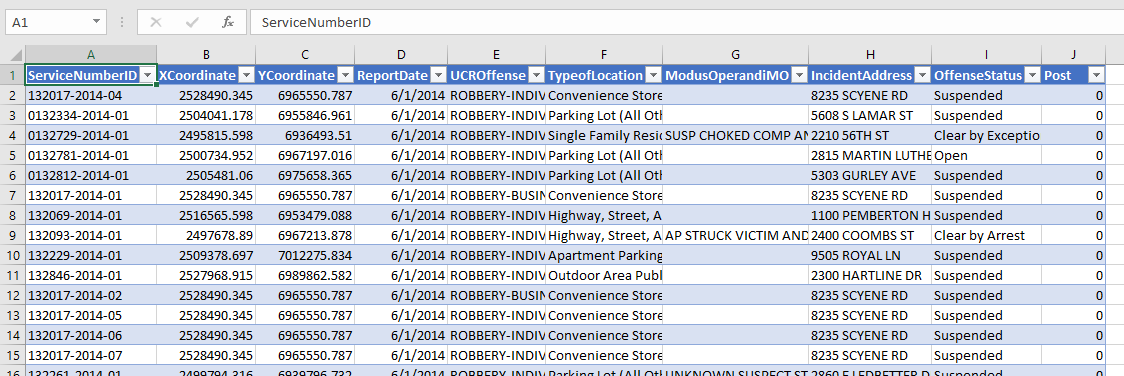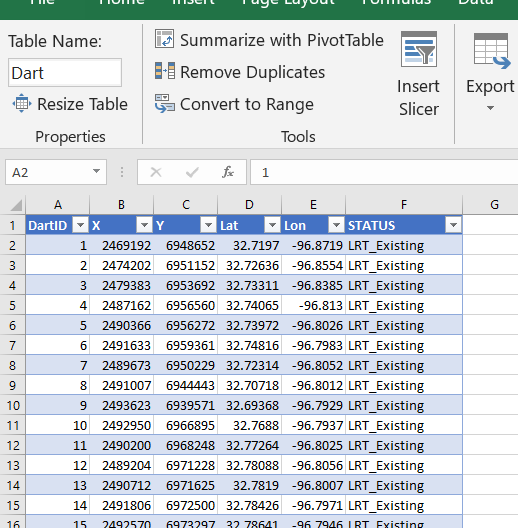# Distance to Nearest

To start, I would typically use a GIS system (or R/python/SQL) to calculate the distance to a nearest object. But I don’t have access to Arc anymore, so I am going to show you a way to do this right in Excel. This only works for projected data (not latitude/longitude), and calculating distance from point-to-point.

So first, to figure out the distance between two points in Euclidean space, we can just use the Pythagorean theorem that you learned in grade school, `Distance = sqrt( (x1 - x2)^2 + (y1 - y2)^2 )`. Because we are doing this in an Excel spreadsheet and want to find the nearest Dart station to the robbery, we will use a little array formula magic. I named my table of Dart locations `Dart`, and so the array formula to find the nearest distance in Excel is:

``=MIN( SQRT( (B2 - Dart[X])^2  + (C2 - Dart[Y])^2))``When you enter this formula, hit `Ctrl` + `Shift` + `Enter`, else it just returns the distance to the first Dart station. If you did this right, you will see the formula have `{}` brackets around it in the formula bar.

Distance will be defined in whatever the projected units are in – here they are in feet. But by using `MIN` with the array, it returns the distance to the nearest station. To get the ID of the associated station, we need to do a similar formula (and this only works with numeric ID fields). You can basically do an array IF formula, and the only station this is true for will be the MAX of that array. (Again hit `Ctrl` + `Shift` + `Enter` when finishing off this cell calculation instead of just `Enter`.)

``=MAX(IF(F2=SQRT((B2 - Dart[X])^2  + (C2 - Dart[Y])^2), Dart[DartID],0))``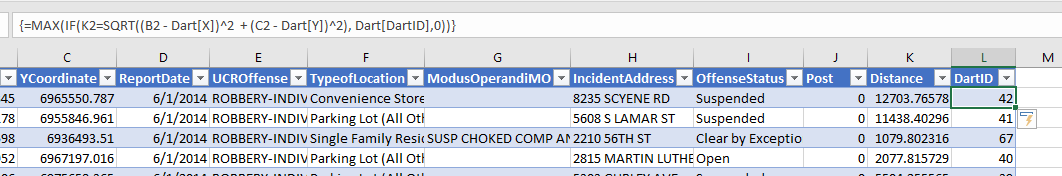User beware – this runs super fast on my machine (surprisingly) but it is quite a few computations under the hood. For much larger data again use a GIS/database/Stat program to do these calculations.

# Using Pivot Tables to do Buffer Analysis

So now that we have those distance fields, it is easy to do a formula along the lines of you want to count up the robberies within 1000 feet. You can do another IF formula that is something like `IF([@Distance] < 1000, 1, 0)`.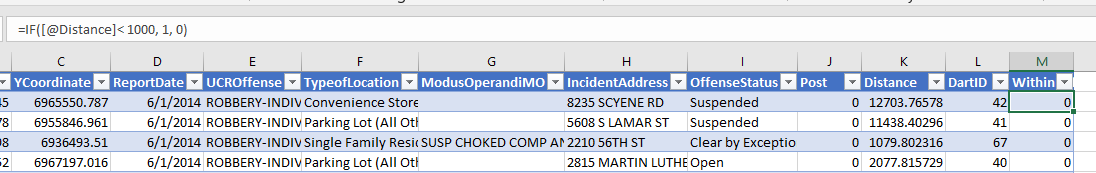And then go ahead and make a pivot table, and put the DartID as the rows, and the Within distance field you just made as the values (to sum in the pivot table).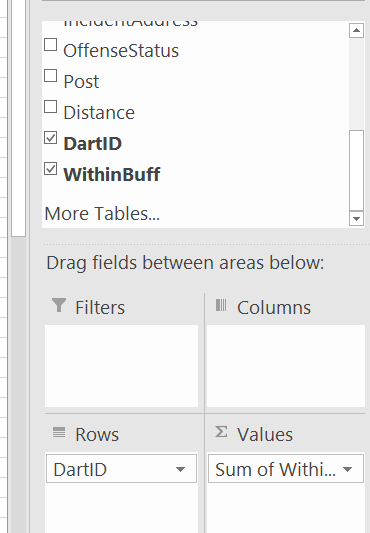Then bam, you have your buffer analysis. Here I sorted the pivot table so you can see the highest crime Dart is 12. (I haven’t looked up which one this is, you can use Excel though to map them out).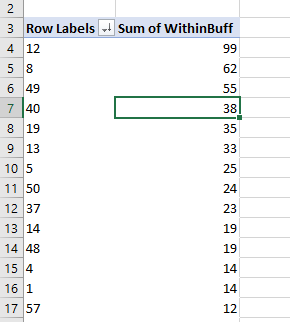So say you wanted to change the buffer size? It is as simple as changing out the `1000` in the prior formula to a different value. One thing I like to do though is to make a lookup table to define different bins. You can see I named that table `BuffTable` (naming the tables makes it easier to refer to them later in array formulas, also I shifted down the pivot table to not accidently overwrite it later).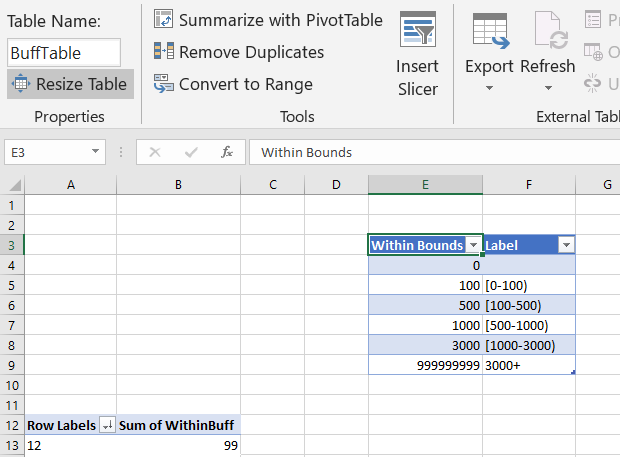And now I use a combination of `MATCH` to find what row it falls into for this table, and `INDEX` to return the row label I want. So first I have `=MATCH([@Distance],BuffTable[Within Bounds],1)`. This is very similar to VLOOKUP, and will match to the row that the distance is less than.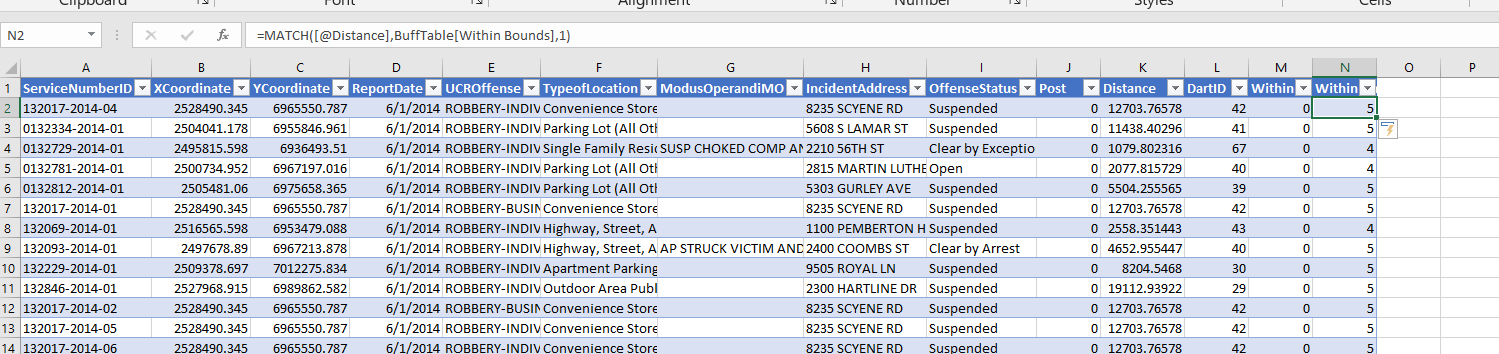This just returns the row number of the match though – I want to pipe in those nicer labels I made. To do that, I nest the match results within index, `=INDEX(BuffTable, MATCH([@Distance],BuffTable[Within Bounds],1)+1, 2)`. And voila, I get my binned data.Now we can do our pivot table so the columns are the new field we just made (make sure to refresh the pivot table).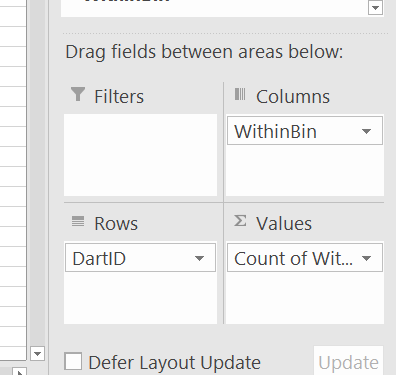And we can do our buffer analysis and varying buffers. Just update the tables to however you want the buffers, hit refresh, and everything will be updated. (I should have done the labels so they are ordered a bit more nicely in the pivot table.)I like this approach for students, as it is easy to pivot/filter on other characteristics as well. Want to get arrest rates around areas? Want to see changes in crimes nearby different DART stations over time? It is just a few formulas/filters and a pivot table away in this format.

# Distance to Nearest Analysis for DART stations

Another analysis I think is useful is to look at the cumulative distance analysis. I got this idea from a paper of Jerry Ratcliffe’s.

So what you can do is to round the distance data, e.g. using a formula like this will round the data to every 300 feet.

``=ROUND([@Distance]/300,0)*300``And then you can make a pivot table of the rounded counts. Here I also did additional stats to calculate the spatial density of the points, and show the distance decay curve.Jerry’s paper I linked to looks for change points – I think that idea is somewhat misleading though. It does look like a change point in Jerry’s graphs, but that is a function of the binning I think (see this Xu/Griffiths paper, same method, finer bins, and shows a more smooth decay).

So here I tied the round function to a cell, and again I can just update the value to a different bin size, and everything get auto-updated in the spreadsheet. Here is a bin size of 100 feet, which introduces some volatility in the very nearby locations, but you can see still pretty much follows that smooth distance decay effect.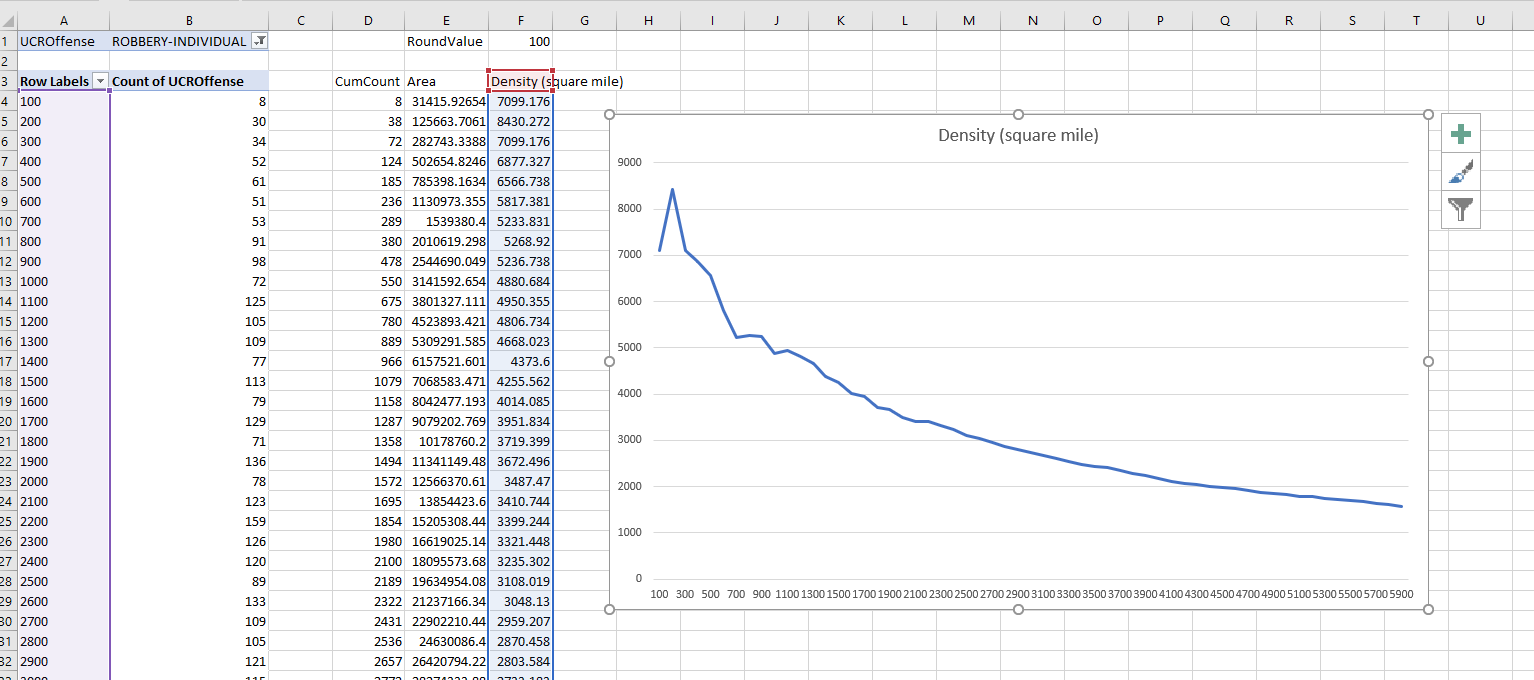Actually the Xu/Griffiths paper looks at the street network distance, which I think makes more sense. (And again need a GIS to do that analysis.) The buffer areas can behave funny, and won’t have a direct relationship to the street length exposure, so I think the typical Euclidean analysis can be misleading in some cases. I will need to save that for another blog post though!